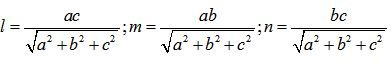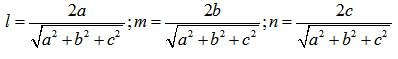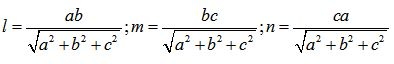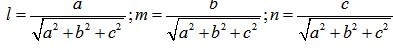# Topic: Three Dimensional Geometry (Test 1)

Topic: Three Dimensional Geometry
Q.1
Direction cosines of a line are
A. The cotangents of the angles made by the line with the negative directions of the coordinate axes.
B. The sines of the angles made by the line with the positive directions of the coordinate axes.
C. The tangents of the angles made by the line with the negative directions of the coordinate axes.
D. The cosines of the angles made by the line with the positive directions of the coordinate axes.
Answer : Option D
Explaination / Solution:

a line when intersect another line or axis two kind of angle form ,as an assumption we take positive side of axis, to define the direction of line we take angle made from all three axis.and we take cos not other trigonometric function like sin,tan because we can define Direction cosines of a line are coefficient of i,j,k of a unit vector along that line.

Workspace
Report
Q.2
If l, m, n are the direction cosines of a line, then
A. 2l2+ m2+ n2= 1.
B.
C. l2+ 2m2+ n2= 1.
D. l2+ m2+ n2= 1.
Answer : Option D
Explaination / Solution:

we know that direction cosines is coefficient of i,j,k of unit vector along that line ,i.e those coefficient are l,m,n .the length of the line r is such that  and magnitute of unit vector r is 1 and . on squaring both side we get
Workspace
Report
Q.3

is a vector joining two points P(x1, y1, z1) and Q(x2, y2, z2).If ,Direction cosines of are

A. x2x1d,y2-y1d,z2z1d
B. x2x1d,y2+y1d,z2z1d
C. x2x1d,y2y1d,z2+z1d
D. x2+x1d,y2y1d,z2z1d
Answer : Option A
Explaination / Solution:

since we know Direction cosines of a line are coefficient of i,j,k of a unit vector along that line,first find a vector   then to convert  it unit vector divide by its magnitute || the coefficient of this unit vector will be
Workspace
Report
Q.4
If l, m and n are the direction cosines of a line, Direction ratios of the line are the numbers which are
A. Inversely Proportional to the direction cosine l of the line
B. Proportional to the direction cosine l of the line
C. Proportional to the direction cosines of the line.
D. Inversely Proportional to the direction cosines of the line
Answer : Option C
Explaination / Solution:

If l, m and n are the direction cosines of a line, Direction ratios of the line are the numbers which are Proportional to the direction cosines of the line.

Workspace
Report
Q.5
If l, m, n are the direction cosines and a, b, c are the direction ratios of a line then
A.B.C.D.Answer : Option D
Explaination / Solution:

using direction ratio a,b,c we got a vector  along  the line ,for direction cosine find  unit vector thenwhere l,m,n represent direction cosine

Workspace
Report
Q.6
Skew lines are lines in different planes which are
A. parallel
B. neither parallel nor intersecting
C. parallel and intersecting
D. intersecting
Answer : Option B
Explaination / Solution:

By definition : The Skew lines are lines in different planes which are neither parallel nor intersecting .

Workspace
Report
Q.7
Angle between skew lines is
A. the angle between two intersecting lines drawn from any point parallel to each of the skew lines
B. the angle between two intersecting lines drawn from any point perpendicular to each of the skew lines
C. the angle between two non intersecting lines drawn from any point parallel to each of the skew lines
D. the angle between two non intersecting lines drawn from any point anti – parallel to each of the skew lines
Answer : Option A
Explaination / Solution:

Angle between skew lines is the angle between two intersecting lines drawn from any point parallel to each of the skew lines .

Workspace
Report
Q.8
If  and  are the direction cosines of two lines; and  is the acute angle between the two lines; then
A.
B. cosθ=|l1m2+m1m2+n1n2|
C. cosθ=|l1l2+m1m2+l1n2|
D. cosθ=|l1l2+l1m2+n1n2|
Answer : Option A
Explaination / Solution:

If  and  are the direction cosines of two lines; and  is the acute angle between the two lines; then the cosine of the angle between these two lines is given by :  .

Workspace
Report
Q.9
If  and  are the direction ratios of two lines and  is the acute angle between the two lines; then
A.B.C.D.Answer : Option A
Explaination / Solution:

If  and  are the direction ratios of two lines and  is the acute angle between the two lines; then , the cosine of the angle between these two lines is given by :Workspace
Report
Q.10
Vector equation of a line that passes through the given point whose position vector is     and parallel to a given vector  is
A.B.C.D.Answer : Option A
Explaination / Solution:

Vector equation of a line that passes through the given point whose position vector is    and parallel to a given vector     is given by :Workspace
Report